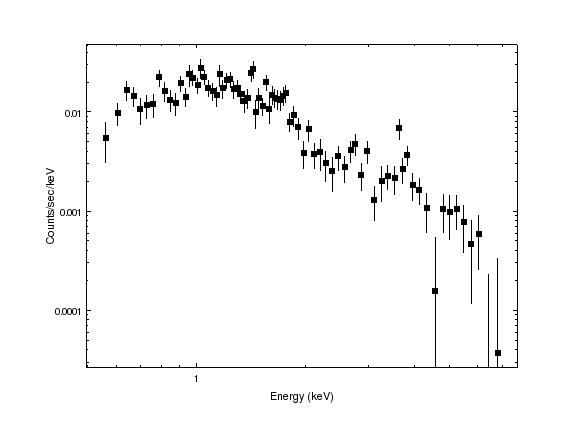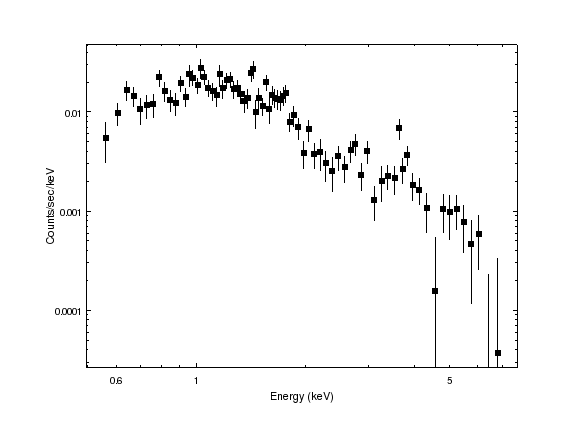## Can I label other numbers than 1 and 10 for a log-scaled axis?

The default labelling of log-scaled axes may not be sufficient for your purposes; for example the Sherpa plot below could do with additional labels on the X axis.The set_arbitrary_tick_positions command would normally be used for this task, but it currently does not work well with logarithmically-scaled axes so we are left with placing the labels ourselves. As labels placed using the data-coordinate system are clipped at the plot boundary, we need to place the labels using a different coordinate system, and to do this, we use the convert_coordinate command to convert from data coordinates such as x=0.6 to plot-normalized coordinates.

chips> convert_coordinate([0.6,1], DATA, PLOT_NORM)
array([ 0.06922294,  0.79010769])


In the above, we have found that the x=0.6 label should be positioned at 0.069 in plot-normalized coordinates. Continuing for our other label locations (0.6, 1, and 5) gives us:

chips> x06 = convert_coordinate([0.6,1], DATA, PLOT_NORM)
chips> x1 = convert_coordinate([1,1], DATA, PLOT_NORM)
chips> x5 = convert_coordinate([5,1], DATA, PLOT_NORM)


and these can be used to create labels by saying

chips> lopt = ["coordsys", PLOT_NORM, "halign", 0.5, "font", "helvetica", "size", 12, "stem", "xlbl"]
chips> info()

Window [win1]
Frame [frm1]
Plot [plot1]   (0.15,0.15)  .. (0.90,0.90)
Border bottom [bx1]  top [bx2]  left [by1]  right [by2]
Curve [crv1]
X Axis [ax1]
Y Axis [ay1]
Label [xlbl1]
Label [xlbl2]
Label [xlbl3]


We also need to hide the existing tick label by saying:

chips> set_xaxis(["ticklabel.visible", True])


The result is:The label font name and size were taken to match those used for the axis:

chips> get_xaxis().ticklabel

angle = 0.0
color = default
font = helvetica
fontstyle = normal
halign = -99.0
offset = 6
size = 12
style = outside
valign = -99.0
visible = False


and the horizontal alignment set to 0.5 so that the labels are centered at the location. the use of a negative y value places the labels outside the plot; the actual value to use depends on the font size and other elements of the visualization. The stem value was set to make these labels easy to distinguish from other labels added to the graph.

Warning: Since the labels are positioned using plot-normalized coordinates then they will not move if the axis is changed by either a limits call or a change of scale (lin_scale or log_scale). Changes to the plot size - using commands like split or reposition_plot - will not invalidate the label positions. It is suggested that these labels only be added once the final layout has been reached.